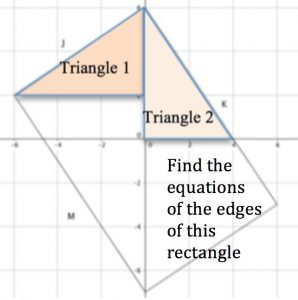A sequence of activities in this guide takes you from familiar ideas about lines, then emphasises the ‘across’ and ‘up’ aspect of gradient to explain why the product of gradients of perpendicular lines is -1, then uses Pythagoras Theorem to produce the equations of circles and recaps on properties of perpendicular lines to produce the equations of tangents.